×
Get Full Access to UH - MATH 3339 - Study Guide - Midterm
Get Full Access to UH - MATH 3339 - Study Guide - Midterm

×

UH / Mathematics / MATH 3339 / What are the types of samples?

# What are the types of samples? Description

##### Description: This study guide covers a review from videos 1-4, vocab, and R studio commands.
10 Pages 214 Views 3 Unlocks
Reviews

Exam 1 Study Guide

## What are the types of samples?Types of Samples:

- Probability sample- a sample in which each member of the population has a chance of being  selected

- Simple Random Sample (SRS)- size n consists of n individuals, each individual of every set  has an equal chance of being selected

- Stratified sampling- divide the population into at least two groups that share the same  characteristics then draw a SRS from each group

- Cluster sampling- divide the population area in clusters, then randomly select clusters, then  randomly select members from those clusters

- Systematic sampling- selecting every k^th member of the population for the sample  - Resampling- many samples are repeatedly taken from available points from the population;  also called bootstrap

## What is quantitative variable?Biased samples

- Biased study- sampling method favors one over the other to get certain outcomes  - Voluntary response sample- people choose to respond in a study/survey and it is biased  towards people who want to respond, people who have strong opinions

- Convenience sampling- chooses samples that are easiest to reach  We also discuss several other topics like What two types of data are used to create phylogenies?

Concepts

- study- an experiment in which we perform something to get a response, gives evidence for  factors causing response & control some of the outcomes

- units=people

- placebo=dummy treatment

- factors=explanatory variables

- placebo effects= subjects responds to placebo treatment

## Define probability.- Random experiments- can be repeated infinite number of times, outcomes vary from  replication even though conditions are the same, each replication is independent meaning  outcomes do not affect the outcomes of others

- Random Variable- variable whose value is a numerical outcome of a random phenomenon  - Categorical Variable- represents data which can be divided into groups  - Quantitative variables- variable on a numerical scale

• Discrete- can count the values, usually whole numbers,

• Continuous- value that can be obtained by measuring, data can take on any value between  two specified values, can be decimals ex:height

- Sample space- a set of all possible outcomes  We also discuss several other topics like If we have a chiral center, how do we know where it points?
We also discuss several other topics like What is the oxfam model?

• Notation for sample space: Ω= {1,2,3,4,5}

- Parameter- a fixed number that describes the population  If you want to learn more check out What is learning & memory in behavioral neuroscience?
If you want to learn more check out What is the meaning of intrinsic in motivation?

- Statistics- a number that describes a sample, known when we have taken a sample  • Multiply or dividing the original data by the same value will change the SD by that factor  (mean & SD change)

• Adding or subtracting the original data by the same value will not change the SD (mean  changes but SD stays the same)

• If all data values are the same, then SD = 0

- Finding standard deviation- average distance each value is from the mean  1. Square each data value

2. Add all squared data values=sum

3. (1/n-1)[sum-(n x mean)= s^2= variance

4. √s^2= SD

- Coefficient of variation- ratio of SD to mean, used to compare variation between two groups  • cv=SD/mean  If you want to learn more check out Who both arrived at the principle of natural selection at the same time?

- p^th percentile- value that p percent of the observation fall at or below it  • nP+0.5

- Five number summary - min, Q1, median, Q3, max

- Q1- 25% of the variable is at that value or less

- Q2- 50% of the variable is at that value or less

- Q3- 75% of the variable is at that value or less

- Interquartile range- range of 50th percentile

• IQR=Q3-Q1

• Q1-(1.5IQR)=A

• Q3+(1.5IQR)=B

• [A,B] anything outside the interval are outliers

- Range= largest value- smallest value

- Mode- most occurring

- Mean- arithmetic average

• sum of all values/# of values

- Center- mena, median, mode

- Range- range, sd, variance, IQR

Graphs

Categorical variable

- Bar graphs- each bar represents a category and the height of each bar are represented by the  count or percent

- Pie charts- helps us see what part of the whole each group forms

Quantitative variable

- Dot plot- dots represent each value over a number line

- Stem plot- stems in a vertical column with the smallest at the top and leaves in the row to the  right of its stem in increasing order out from the stem

- Histogram- like bar graphs for quantitative variable, bars touching, width of bar represents a  range of values, height of bar represents number of cases within that range of values  - Boxplot- represents the five number summary, useful for side by side comparison, asterisks or  dots represent outliers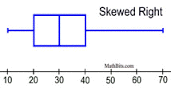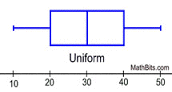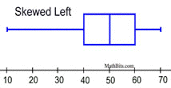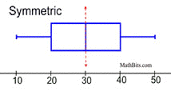- Cumulative frequency polygon- y axis has percentile and x- axis has the variable, graph can  show values at each percentile

- Distributions- tells us what values it takes and how often it takes these values based on the  individuals, COSS

• Center- median/center

• Outliers- values outside the overall pattern

• Shape- symmetric, skewed right, skewed left, uniform

• Spread- largest value- smallest value

Probability

- Random if individual outcomes are uncertain

- Scatterplots- show association between two quantitative variables

• Direction- positive= increasing, negative=decreasing

• Form- if there is a straight line relationship= linear

• Strength- how much scatter the plot has, very strong, moderate, or weak association  - Set- collection of objects

- Elements- individual elements in a set

• E= {….,….}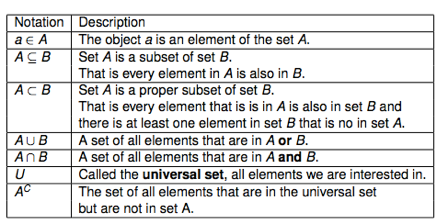- Venn diagrams- show relationships between two sets

- Classical methods- probability of all outcomes are equal

• 1/n to each possible outcome

- Relative frequency method- using data to estimate proportion of the time the outcome will  occur in the future

• p(A)= #of times A occurs/tota #of observations

- Subjective method- assigning probability known possible outcomes do not have equal  probability and little data is known

- Tree diagram- graphical representation in visualizing a multiple step experiment  - 0!=1

- Permutations- computing number of outcomes where order does matter  • nPr= (n!)/(n-r)!

• P= (n!)/[(r!)(s!)(t!)] for repeating letters

• Circular permutations- (n-1)!

- Combinations- counts number of outcomes where order doesn’t matter  • nCr=(n!)/(r!(n-r)!)

- Probability Rules

1. P(E) has to be between 0 and 1

2. P(Ω)= 1

3. There are no elements in A&B= pairwise disjoint

4. Complement Rule- P(~A)= 1-P(A)

5. P(Ø)= O no elements

6. Addition Rule- P(AuB)= P(A)+P(B)-P(AnB) when finding the chance of events A or B  happening

7. Multiplication- P(AnB)= P(A) x P(B, given A) OR P(AnB)= P(B) x P(A, given B) when  finding the chance of events of A and B happening

8. Conditional probability- P(A,given B)=(P(AnB))/(P(B))

R studio

- Type the following to make a list of values - dataname=c(data,data,data)  - To import data, go to tools, click on import dataset, upload by URL or local file  - If data is already in R, then type the data name and click enter

- To find mean, median, variance, sd of the data

• if it has one variable- mean(dataname)

• if it has multiple variables- mean(dataname\$variable)

• if it has one variable- median(datasetname)

• if it has multiple variables- median(dataname\$variable)

• if it has one variable- var(dataname)

• if it has multiple variables- var(dataname\$variable)

• if it has one variable- sd(datasetname)

• if it has multiple variables- sd(dataname\$variable)

- To find percentile

• quantile(dataname,enter percentile in decimal) ex:(KidsFeet,0.25,0.50)  - To find five number summary

• fivenum(dataname)

- Bar graph- plot(dataname\$variable)

- Pie chart-

• First type- counts<- table(dataname\$variable) press enter

• Then type- pie (counts)

- Stem and Leaf plot- stem(dataname\$variable)

- Histogram- hist(dataname\$variable)

• To plot a histogram with a title and x-axis label

hist(dataname4variable,main=“Title”,xlab=“x-axislabel”

- Boxplot- boxplot(dataname\$variable)

• To plot boxplots side by side- use tilde as shown below

• boxplot(quantitativevariable\$categoricalvariable~quantitativevariable\$categoricalvariable)  - Scatterplot- plot(explanatory, response)

- For permutations- factorial(n)

- For combinations- choose(n,r)

Exam 1 Study Guide

Types of Samples:

- Probability sample- a sample in which each member of the population has a chance of being  selected

- Simple Random Sample (SRS)- size n consists of n individuals, each individual of every set  has an equal chance of being selected

- Stratified sampling- divide the population into at least two groups that share the same  characteristics then draw a SRS from each group

- Cluster sampling- divide the population area in clusters, then randomly select clusters, then  randomly select members from those clusters

- Systematic sampling- selecting every k^th member of the population for the sample  - Resampling- many samples are repeatedly taken from available points from the population;  also called bootstrap

Biased samples

- Biased study- sampling method favors one over the other to get certain outcomes  - Voluntary response sample- people choose to respond in a study/survey and it is biased  towards people who want to respond, people who have strong opinions

- Convenience sampling- chooses samples that are easiest to reach

Concepts

- study- an experiment in which we perform something to get a response, gives evidence for  factors causing response & control some of the outcomes

- units=people

- placebo=dummy treatment

- factors=explanatory variables

- placebo effects= subjects responds to placebo treatment

- Random experiments- can be repeated infinite number of times, outcomes vary from  replication even though conditions are the same, each replication is independent meaning  outcomes do not affect the outcomes of others

- Random Variable- variable whose value is a numerical outcome of a random phenomenon  - Categorical Variable- represents data which can be divided into groups  - Quantitative variables- variable on a numerical scale

• Discrete- can count the values, usually whole numbers,

• Continuous- value that can be obtained by measuring, data can take on any value between  two specified values, can be decimals ex:height

- Sample space- a set of all possible outcomes

• Notation for sample space: Ω= {1,2,3,4,5}

- Parameter- a fixed number that describes the population

- Statistics- a number that describes a sample, known when we have taken a sample  • Multiply or dividing the original data by the same value will change the SD by that factor  (mean & SD change)

• Adding or subtracting the original data by the same value will not change the SD (mean  changes but SD stays the same)

• If all data values are the same, then SD = 0

- Finding standard deviation- average distance each value is from the mean  1. Square each data value

2. Add all squared data values=sum

3. (1/n-1)[sum-(n x mean)= s^2= variance

4. √s^2= SD

- Coefficient of variation- ratio of SD to mean, used to compare variation between two groups  • cv=SD/mean

- p^th percentile- value that p percent of the observation fall at or below it  • nP+0.5

- Five number summary - min, Q1, median, Q3, max

- Q1- 25% of the variable is at that value or less

- Q2- 50% of the variable is at that value or less

- Q3- 75% of the variable is at that value or less

- Interquartile range- range of 50th percentile

• IQR=Q3-Q1

• Q1-(1.5IQR)=A

• Q3+(1.5IQR)=B

• [A,B] anything outside the interval are outliers

- Range= largest value- smallest value

- Mode- most occurring

- Mean- arithmetic average

• sum of all values/# of values

- Center- mena, median, mode

- Range- range, sd, variance, IQR

Graphs

Categorical variable

- Bar graphs- each bar represents a category and the height of each bar are represented by the  count or percent

- Pie charts- helps us see what part of the whole each group forms

Quantitative variable

- Dot plot- dots represent each value over a number line

- Stem plot- stems in a vertical column with the smallest at the top and leaves in the row to the  right of its stem in increasing order out from the stem

- Histogram- like bar graphs for quantitative variable, bars touching, width of bar represents a  range of values, height of bar represents number of cases within that range of values  - Boxplot- represents the five number summary, useful for side by side comparison, asterisks or  dots represent outliers- Cumulative frequency polygon- y axis has percentile and x- axis has the variable, graph can  show values at each percentile

- Distributions- tells us what values it takes and how often it takes these values based on the  individuals, COSS

• Center- median/center

• Outliers- values outside the overall pattern

• Shape- symmetric, skewed right, skewed left, uniform

• Spread- largest value- smallest value

Probability

- Random if individual outcomes are uncertain

- Scatterplots- show association between two quantitative variables

• Direction- positive= increasing, negative=decreasing

• Form- if there is a straight line relationship= linear

• Strength- how much scatter the plot has, very strong, moderate, or weak association  - Set- collection of objects

- Elements- individual elements in a set

• E= {….,….}- Venn diagrams- show relationships between two sets

- Classical methods- probability of all outcomes are equal

• 1/n to each possible outcome

- Relative frequency method- using data to estimate proportion of the time the outcome will  occur in the future

• p(A)= #of times A occurs/tota #of observations

- Subjective method- assigning probability known possible outcomes do not have equal  probability and little data is known

- Tree diagram- graphical representation in visualizing a multiple step experiment  - 0!=1

- Permutations- computing number of outcomes where order does matter  • nPr= (n!)/(n-r)!

• P= (n!)/[(r!)(s!)(t!)] for repeating letters

• Circular permutations- (n-1)!

- Combinations- counts number of outcomes where order doesn’t matter  • nCr=(n!)/(r!(n-r)!)

- Probability Rules

1. P(E) has to be between 0 and 1

2. P(Ω)= 1

3. There are no elements in A&B= pairwise disjoint

4. Complement Rule- P(~A)= 1-P(A)

5. P(Ø)= O no elements

6. Addition Rule- P(AuB)= P(A)+P(B)-P(AnB) when finding the chance of events A or B  happening

7. Multiplication- P(AnB)= P(A) x P(B, given A) OR P(AnB)= P(B) x P(A, given B) when  finding the chance of events of A and B happening

8. Conditional probability- P(A,given B)=(P(AnB))/(P(B))

R studio

- Type the following to make a list of values - dataname=c(data,data,data)  - To import data, go to tools, click on import dataset, upload by URL or local file  - If data is already in R, then type the data name and click enter

- To find mean, median, variance, sd of the data

• if it has one variable- mean(dataname)

• if it has multiple variables- mean(dataname\$variable)

• if it has one variable- median(datasetname)

• if it has multiple variables- median(dataname\$variable)

• if it has one variable- var(dataname)

• if it has multiple variables- var(dataname\$variable)

• if it has one variable- sd(datasetname)

• if it has multiple variables- sd(dataname\$variable)

- To find percentile

• quantile(dataname,enter percentile in decimal) ex:(KidsFeet,0.25,0.50)  - To find five number summary

• fivenum(dataname)

- Bar graph- plot(dataname\$variable)

- Pie chart-

• First type- counts<- table(dataname\$variable) press enter

• Then type- pie (counts)

- Stem and Leaf plot- stem(dataname\$variable)

- Histogram- hist(dataname\$variable)

• To plot a histogram with a title and x-axis label

hist(dataname4variable,main=“Title”,xlab=“x-axislabel”

- Boxplot- boxplot(dataname\$variable)

• To plot boxplots side by side- use tilde as shown below

• boxplot(quantitativevariable\$categoricalvariable~quantitativevariable\$categoricalvariable)  - Scatterplot- plot(explanatory, response)

- For permutations- factorial(n)

- For combinations- choose(n,r)

Page ExpiredIt looks like your free minutes have expired! Lucky for you we have all the content you need, just sign up here